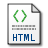# Simulating ruin probabilities for Markov-Additive processes by importance sampling methods

Martinelli, A. (2004) Simulating ruin probabilities for Markov-Additive processes by importance sampling methods. Technical Report. CNR. Istituto di Matematica Applicata e Tecnologie Informatiche (IMATI). Sezione di Milano, Milano, IT. (Unpublished)HTML 04-16.html Restricted to Repository staff only Download (1kB)

## Abstract

In this paper we model a risk process, which starts from some positive level, by means of a Markov additive process with an underlying finite state Markov chain. We aim at evaluating the ruin probability corresponding to such processes by developing a suitable importance sampling algorithm. The ruin probability turns out to be a large deviations probability (as the initial level of the reserve diverges) under fairly general conditions. Given this, we determine an importance sampling measure and show it to be the only asymptotically efficient measure in a wide class of importance sampling measures

Item Type: Monograph (Technical Report) Markov additive processes; Large deviations theory; Ruin probability; Monte Carlo simulation 500 Scienze naturali e Matematica > 510 Matematica biblioteca 3 15 Mar 2006 20 May 2010 12:00 http://eprints.bice.rm.cnr.it/id/eprint/139View Item